# Ranking movies using Bayesian Average

Almost every single website, app or platform on the internet has some sort of rating system in place. Whenever you purchase a product or use a service, you are asked to rate it on a scale, say 1 to 5. The platform then uses this data to generate a score and build a ranking system around it. The score is the measure of quality for each product or service. By surfacing the most quality content on top of the list, the platform tries to up their sales and ensure better engagement with their users.

Coming up with an aggregated score is not an easy thing - we need to crunch a million ratings and then see that the score is, in fact, the true measure of quality. If it isn't then it would directly affect the business. Today we discuss how we should define this score in a rating based system; spoiler alert! the measure is called Bayesian Average.

To keep things simple we define the problem statement as

Given the ratings, on a scale of 1 to 5, that users give to a movie, we generate a score that is a measure of how good a movie is which then helps us get the top 10 movies of all time.

We will use the MovieLens Dataset to explore various scoring functions in this article. In the dataset, we get user ratings for each movie and the ratings are made on a scale of 1 to 5.

# Generating the score

The score we generate for each item should be proportional to the quality quotient which means higher the score, superior is the item. Hence we say that the score of an item is the function of all the `m` ratings that it received.## Arithmetic Mean

The simplest and the most common strategy to compute this aggregated score for an item is by taking an Arithmetic Mean (average) of all the ratings it received. Hence for each item we sum all the ratings that it received and divide it by its cardinality, giving us the average value.### Issues with arithmetic mean

The arithmetic mean falls apart pretty quickly. Let's say there is an item with just one rating of 5 on 5, the item would soar high on the leaderboard ranking. But does it deserve that place? probably not. Because of low cardinality (number of ratings), the score (and hence the rank) of the item will fluctuate more and will not give a true measure of quality.

With the movie dataset, we are analyzing here are the top 10 movies ranked using Arithmetic Mean.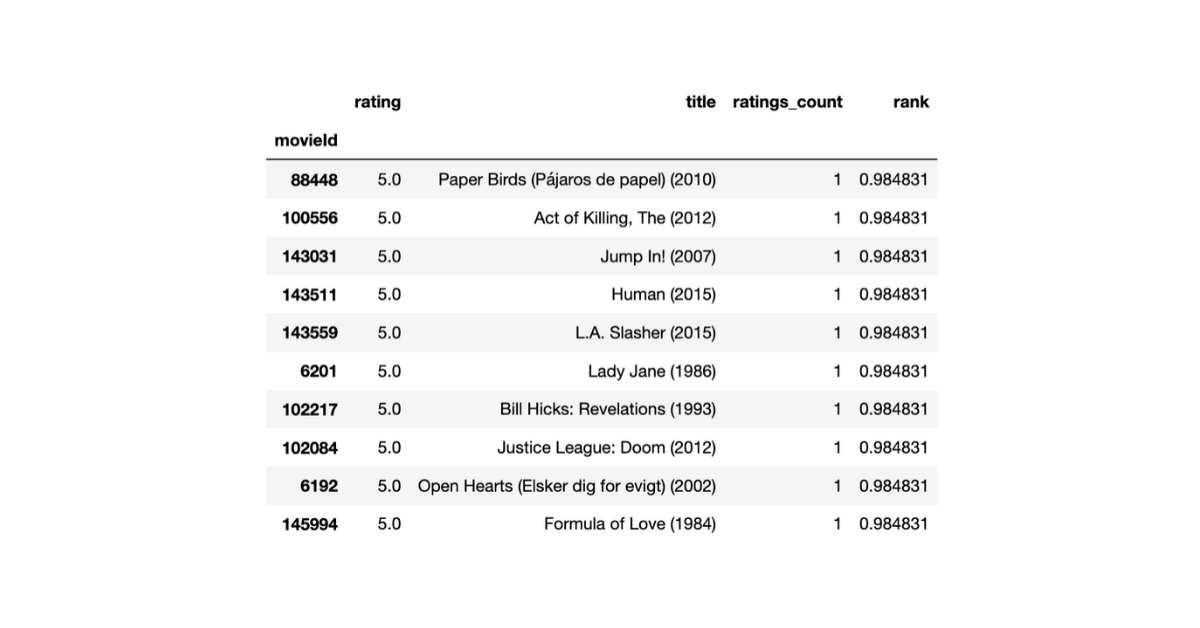Through this measure, all of the top 10 movies have a score of 5 (out of 5) and all of them have just 1 rating. Are these really the top 10 movies of all time? Probably not. Looks like we need to do a lot better than the Arithmetic Mean.

## Cumulative Rating

To remedy the issue with Arithmetic Mean, we come up with an approach of using Cumulative Rating as the scoring function hence instead of taking the average we only consider the sum of all the ratings as the final score.Cumulative Rating actually does a pretty decent job, it makes popular items with a large number of ratings bubble up to the top of the leaderboard. When we rank the movies in our dataset using Cumulative Ratings we get the following as the top 10.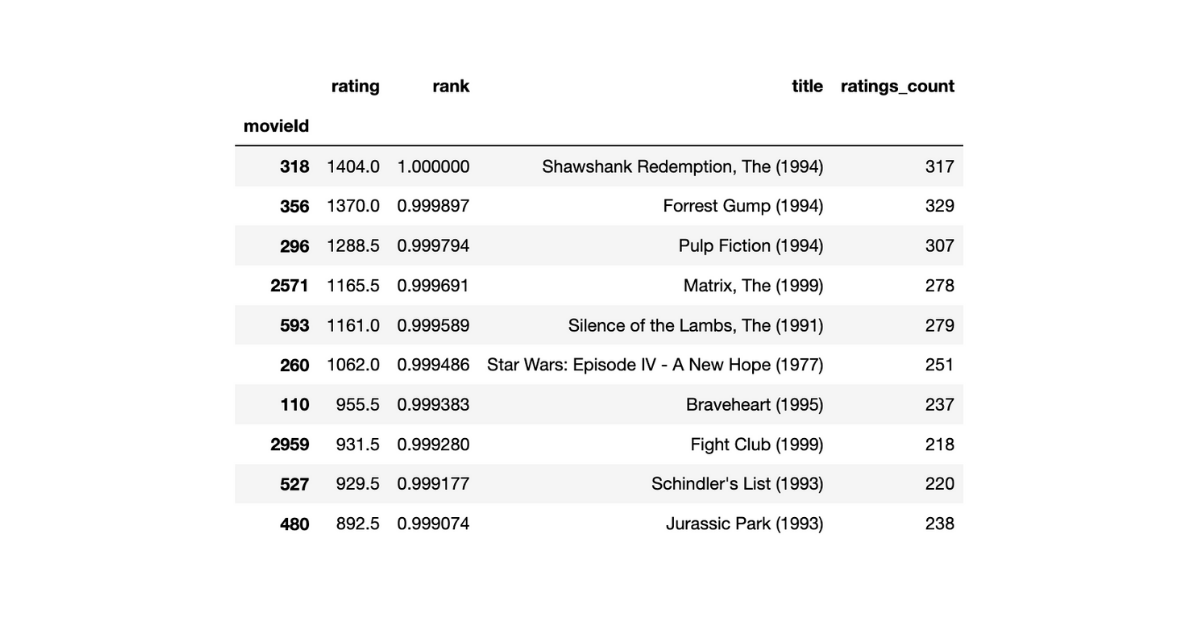The top 10 movies now feature Shawshank Redemption, Forrest Gump, Pulp Fiction, etc. which are in fact considered as the top movies of all times. But is Cumulative Rating fool-proof?

### Issues with cumulative rating

Cumulative Rating favors high cardinality. Let's say there is an extremely poor yet popular item `A` that got 10000 ratings of 1 on 5, and there is another item `B` which is very good but it got 1000 rating of 5 on 5 Cumulative Rating thus gives a score of 10000 * 1 = 10000 to item `A` and 1000 * 5 = 5000 to item `B`, but `B` clearly is far superior of an item than `A`.

Another issue with Cumulative Rating is the fact that it generates an unbounded score. Ideally, any ranking system expects a normalized bounded score so that the system becomes predictable and consistent.

We established that Cumulative Rating is better than Arithmetic Mean but it is not fool-proof and that's where the Bayesian Average comes to the rescue.

# The Bayesian Average

Bayesian Average computes the mean of a population by not only using the data residing in the population but also considering some outside information, like a pre-existing belief - a derived property from the dataset, for example, prior mean.

## The intuition

The major problem with Arithmetic Mean as the scoring function was how unreliable it was when we had a low number of data points (cardinality) to compute the score. Bayesian Average plays a part here by introducing pre-belief into the scheme of things.

We start by defining the requirements of our scoring function

• for an item with a fewer than average number of ratings - the score should be around the system's arithmetic mean
• for an item with a substantial number of ratings - the score should be the item's arithmetic mean
• as the number of ratings that an item receives increases, the score should gradually move from system's mean to item's mean

By ensuring the above we neither prematurely promote nor demote an item in the leaderboard. An item is given a fair number of chances before its score falls to its own Arithmetic mean. This way we use the prior-belief - System's Arithmetic mean, to make the scoring function more robust and fair to all items.

## The formula

Given the intuition and scoring rules, we come up with the following formulaIn the above formula, `w` indicates the weight that needs to be given the item's Arithmetic Mean `A` while `S` represents the System's Arithmetic Mean. If `A` and `S` are bounded then the final score `s` will also be bounded in the same range, thus solving the problem with Cumulative Rating.

Suppose the number of ratings that an item `i` receives is denoted by `m` and the average number of ratings that any item in the system receives is denoted by `m_avg`, we define the requirements of weight `w` as follows

• `w` is bounded in the range [0, 1]
• `w` should be monotonically increasing
• `w` should be close to 0 when `m` is close to 0
• `w` should reach 0.5 when number `m` reaches `m_avg`
• `w` tries to get closer to 1 as `m` increases

From the above requirements, it is clear that `w` is acting like a knob which decides in what proportions we should consider an item's mean versus the system's mean. As `w` increases we tilt more towards item's mean. We define the `w` asWhen we combine all of the above we get the final scoring function as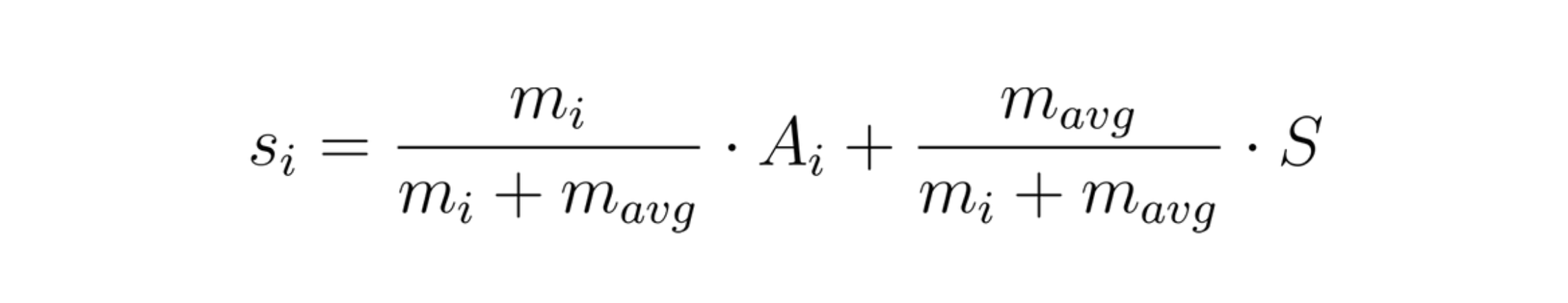One of the most important properties of Bayesian Average is the fact that the pre-existing belief acts as support which oversees that the score does not fluctuate too abruptly and it smoothens with more number of ratings.

## Applying Bayesian Average to movies dataset

After applying the above mentioned Bayesian Average scoring function to our Movie dataset, we get the following movies as top 10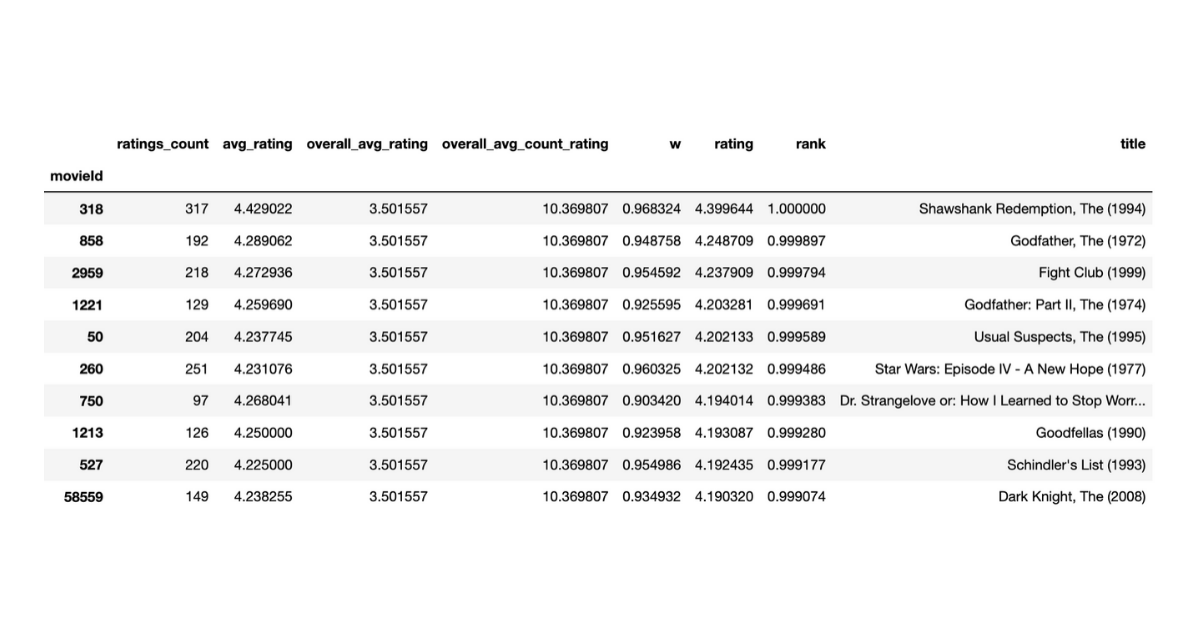Pretty impressive list! The list contains almost all the famous movies that we all think make the cut. Bayesian average thus provides a bounded score that is a measure of the quality of the item, by using prior-belief i.e. system's mean.

## Analyzing how Bayesian Average changes the rank

Now that we have seen that the Bayesian Average is, in fact, an excellent way to rank items in a rating system, we find how the rank of an item changes as it receives more ratings. Below we plot the change in the percentile rank of the movies: Kingsman, Logan and The Scorpion King.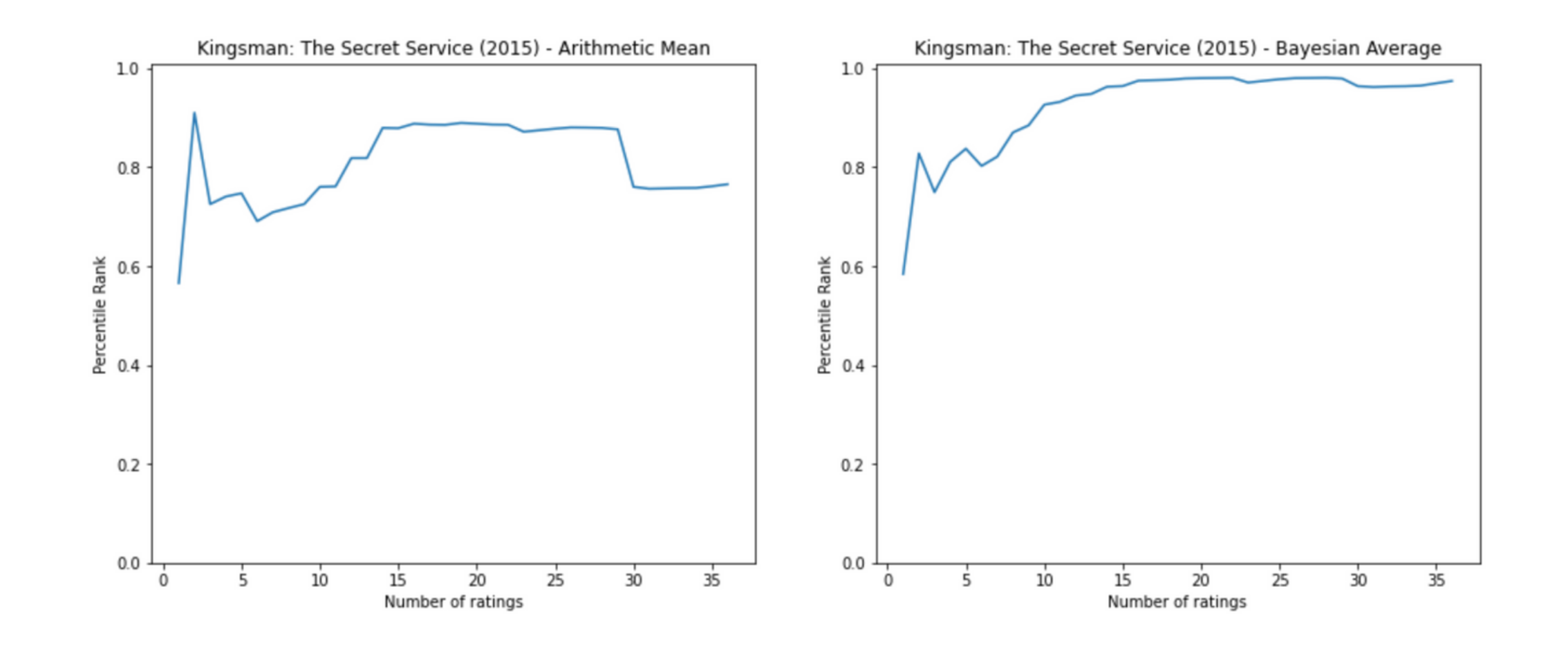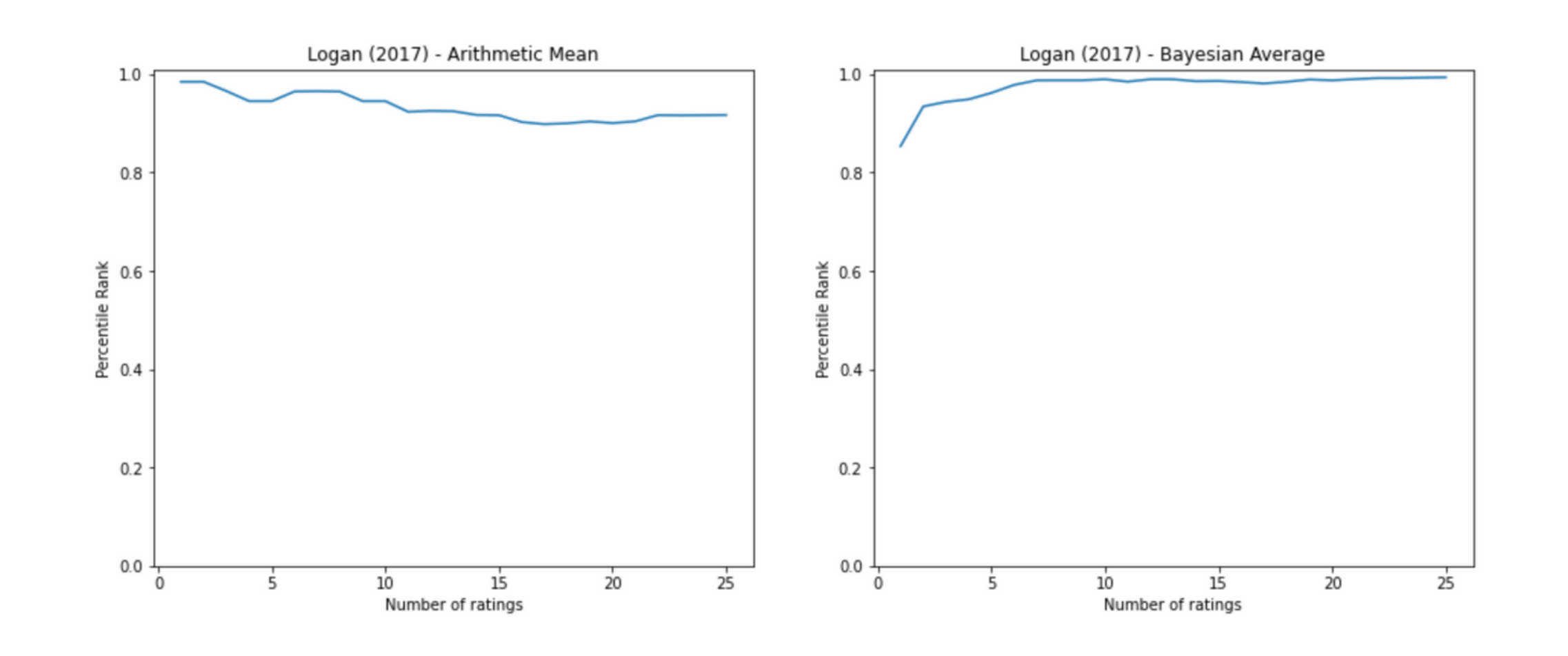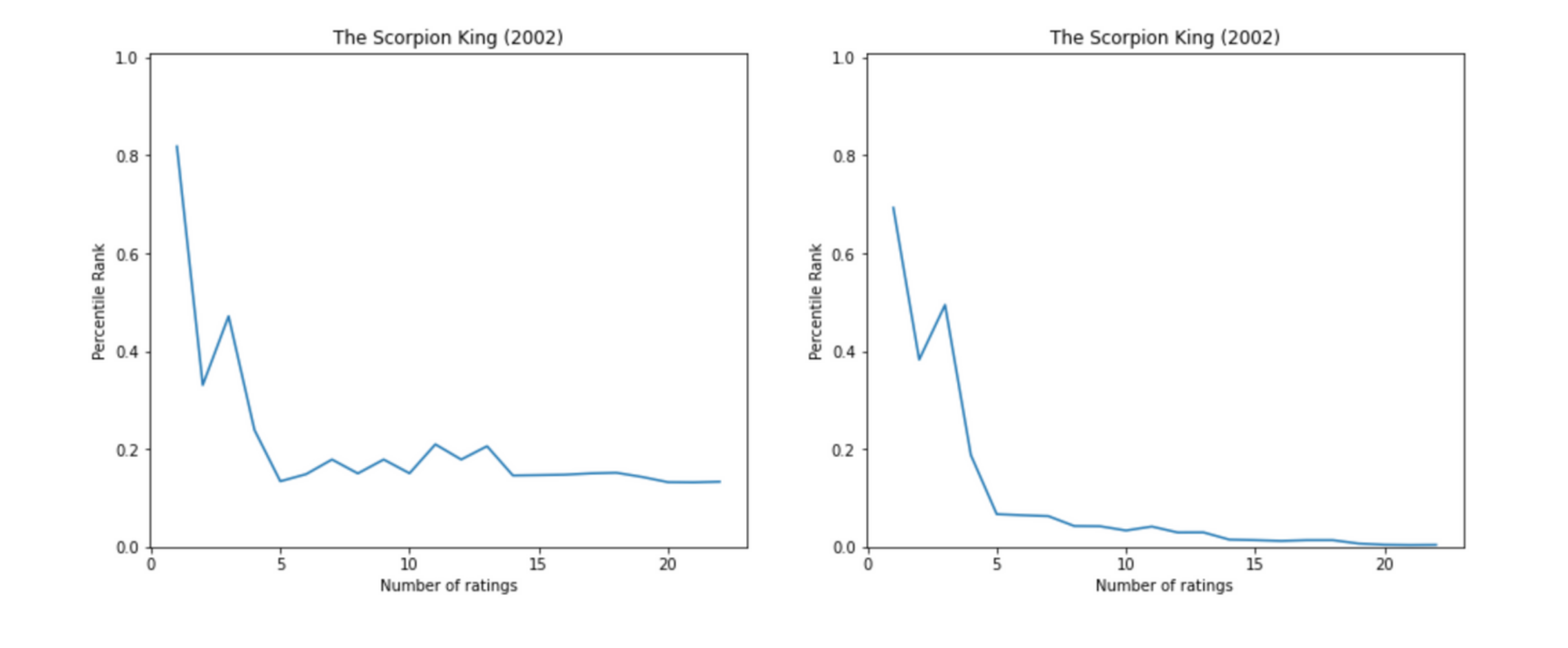We observe that the fluctuations in percentile rank are more in the case of Arithmetic Mean. Sometimes even after receiving a good number of reviews, the rank fluctuates sharply. In the case of Bayesian Average after an initial set of aberrations, the rank smoothens and converges.

# A note on Bayesian Average

Bayesian Average is not a fixed formula that we have seen above, but it is a concept where we make the scoring function "smoother" by using a pre-existing belief as support. Hence we can tweak the formula as per our needs, or use multiple prior beliefs and still it would classify as a Bayesian Average.

As submitted by author, the post was originally published here.

# Arpit Bhayani

Principal Engineer @Unacademy • Data @Amazon • Platform @Practo | Writes about Language internals, System Design, Programming and Math in Computer Science.

Arpit has published 13 articles so far